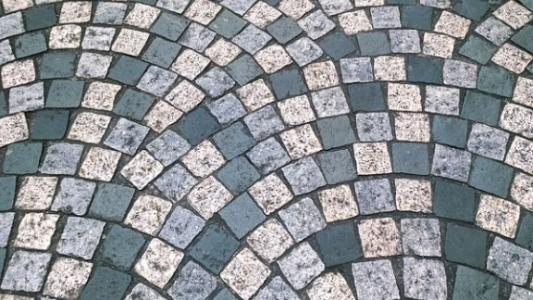# Number Bases

We count in base ten because most of us have ten fingers. But what if we only had seven or eight? Here's a chance for you to explore the properties of base 10 as well as some less familiar number bases.### Measuring with Rods

How many different lengths is it possible to measure with a set of three rods? Explore this problem, and make some discoveries about number bases.### Always a Multiple?

Think of a two digit number, reverse the digits, and add the numbers together. Something special happens... Watch the videos and adapt the method to explain a variety of number tricks.### Weights

Imagine you had a set of positive and negative "weights", which can be combined to make different totals. Which totals can you make?